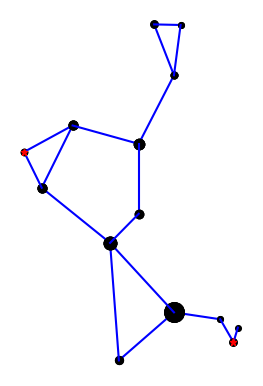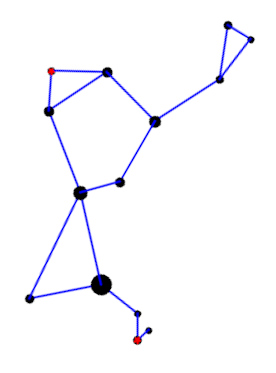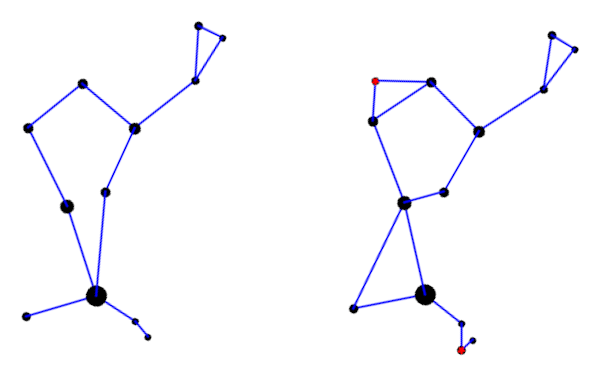# Two ways of connecting the stars in Boötes (Herdsman)

A couple weeks ago I wrote about how H. A. Rey introduced a new way of looking at the constellations, making them look more like their names. That post used Leo as an example. This post looks at Boötes (The Herdsman) .

Here is the constellation using the connections indicated in the IAU star chart.Here is the constellation using the connections drawn in Rey’s book .Rey’s version adds two stars, highlighted in red, but mostly connects the same stars in a different way. I suppose the herdsman is standing in the IAU version; it’s hard to tell. In Rey’s version, the huntsman is clearly seated and smoking a pipe. This is easier to see if we rotate the image a bit.Here’s a comparison of the two interpretations side-by-side.Here is the Python code that produced the two images. It’s a little cleaner than the code in the earlier post, and it draws larger dots to represent brighter stars.

```import matplotlib.pyplot as plt

# data from https://en.wikipedia.org/wiki/List_of_stars_in_Bo%C3%B6tes

α = (14 + 16/60, 19 + 11/60, 0.0)
β = (15 +  2/60, 40 + 23/60, 3.5)
γ = (14 + 32/60, 38 + 18/60, 3.0)
δ = (15 + 16/60, 33 + 19/60, 3.5)
ε = (14 + 45/60, 27 +  4/60, 2.3)
ζ = (14 + 41/60, 13 + 44/60, 3.8)
η = (13 + 55/60, 18 + 24/60, 4.5)
θ = (14 + 25/60, 51 + 51/60, 4.0)
κ = (14 + 13/60, 51 + 47/60, 4.5)
λ = (14 + 16/60, 46 +  5/60, 4.2)
μ = (15 + 24/60, 37 + 23/60, 4.3)
υ = (13 + 49/60, 15 + 48/60, 4.0)
τ = (13 + 47/60, 17 + 27/60, 4.5)
ρ = (14 + 32/60, 30 + 22/60, 3.6)

k = -15 # reverse and scale horizontal axis

def plot_star(s, m):
plt.plot(k*s, s, m, markersize=14-2.2*s)

def join(s0, s1, m='ko'):
plot_star(s0, m)
plot_star(s1, m)

plt.plot([k*s0, k*s1], [s0, s1], 'b-')

def draw_iau():

join(α, η)
join(η, τ)
join(α, ζ)
join(α, ϵ)
join(ϵ, δ)
join(δ, β)
join(β, γ)
join(γ, λ)
join(λ, θ)
join(θ, κ)
join(κ, λ)
join(γ, ρ)
join(ρ, α)

def draw_rey():

join(α, η)
join(η, υ)
join(υ, τ)

join(α, ζ)
join(α, ϵ)
join(ζ, ϵ)

join(ϵ, δ)
join(δ, β)
join(δ, μ)
join(μ, β)
join(β, γ)
join(γ, λ)
join(λ, θ)
join(θ, κ)
join(κ, λ)
join(γ, ρ)
join(ρ, ϵ)

plot_star(μ, 'r*')
plot_star(υ, 'r*')
return

draw_iau()
plt.gca().set_aspect('equal')
plt.axis('off')
plt.savefig("bootes_iau.png")
plt.close()

draw_rey()
plt.gca().set_aspect('equal')
plt.axis('off')
plt.savefig("bootes_rey.png")
plt.close()
```

***

 The diaeresis over the second ‘o’ in Boötes means the two vowels are to be pronounced separately: bo-OH-tes. You may have seen the same pattern in Laocoön or oogenesis. The latter is written without a diaresis now, but I bet authors used to write it with a diaeresis on the second ‘o’.

 H. A. Rey. The Stars: A New Way to See Them, Second Edition.

Source: https://www.johndcook.com/blog/2023/03/26/bootes/
#ways #connecting #stars #Boötes #Herdsman